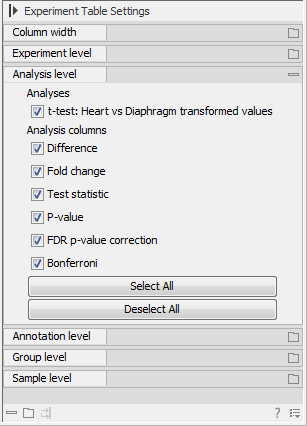### Analysis level

The results of each statistical test performed are in the columns listed in this area. In the table, a heading is given for each test. Information about the results of statistical tests are described in the Statistical analysis.

An example of Analysis level settings is shown in figure 31.7.Figure 31.7: An example of columns available under the Analysis level section.

Note: Some column names here are the same as ones under the Experiment level, but the results here are from statistical tests, while those under the Experiment level section are calculations carried out directly on the expression levels.